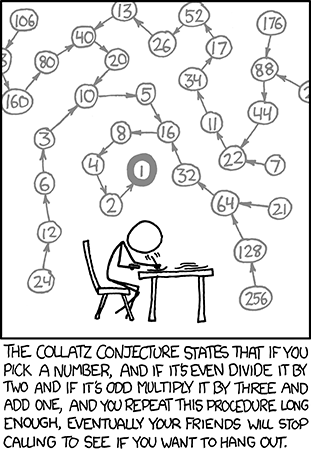## Collatz Problem a.k.a. 3n+1 Problem

This post focuses on Collatz problem, which is also known as, among others, the 3n+1 problem, and  the Syracuse problem.

Outline. We begin by introducing Collatz conjecture; afterwards, we presents an algorithm to solve theproblem (UVa 100 or SPOJ 4073) published in both UVa and SPOJ. The primary advantage of having it in SPOJ is that we can use F# to derive a simple and elegant solution; at the same time, we can verify it via SPOJ’s online judge.

# Background: Collatz Conjecture

Collatz problem, also known asproblem, concerns the iterates generated by the repeated applications of following the function: Given a positive integer,which implies that ifis even, it returns, otherwise. This function is called Collatz function. Consider, for instance,, the generated iterates are following:. This sequence is referred to as Collatz sequence, or hailstone numbers.Collatz conjecture, which is credited to Luther Collatz at the University of Hamburg, asserts that for any positive integer, the repeated applications of the Collatz function, i.e.,eventually produces value; that is,, wheredenotesapplication of. Considering, it follows:.

The generated sequence of iterates:is called the Collatz-trajectory of. For instance, beginning from, the resulted sequence converged toas follows:. Therefore, Collatz-trajectory of 26 is. Note that, although Collatz problem is based on this simple concept, it is intractably hard. So far, it has been verified forby Leaven and Vermeluen.

# Algorithm Design: 3n+1 problem

In the rest of this post, we intend to solve theproblem, which is essentially a restricted or bounded version of the Collatz problem.

Interpretation. It restricts the iteration by exiting as soon as the Collatz sequence reaches to value 1 at. For= 26, the resulting Collatz sequence is therefore:, and length of the sequence isi.e., 11. This problem asks to compute the largest Collatz sequence that results from any integer betweenand, which are provided as inputs. Note that, the value ofandare both positive integers:.

Implementation. We first apply a naïve brute-force algorithm to solve this problem, which computes the length of the sequence for each integer fromto, and returns the maximum length found.  It is worth noting that-A naïve brute-force algorithm redundantly computes sequences again and again. Consider=13 and=26. For= 26, we also compute the sequence for 13 that has already  been computed during= 13.We must apply a tail-recursive implementation to compute the sequence, as naïve implementation might results in stack overflow.

As we shall see next, we have optimized the naïve implementation considering the above observations. First, we define function, `nextCollatz`, that returns next integer of the Collatz sequence, given an integer. In effect, it computesfromas follows.

Using the algorithm outlined in `collatzSeqLength`, the length of the Collatz sequence is computed for any given integer.

It includes the following optimizations over the naïve implementation we stated earlier:Memorization has been incorporated to effectively optimize the algorithm by avoiding redundant computations of the sequence and its length, which in turn provides a faster algorithm than its naïve counterpart, albeit with the cost of additional space.Tail-recursive algorithm enables computation of Collatz sequence with larger.Continuation-passing-style  has been applied in this algorithm to accommodate, and to combine tail-call optimization with memorization.

The following snippet demonstrates how the maximum sequence length is obtained by invoking collatzSeqLength for each integer between the givenand.

Complete source code of this problem can be found in this gist. Following IDEONE page (with sample inputs and outputs) has been provided to further play with the code (in case of the unavailability of F# in local system).  Java source code is also available for this problem.

Try solving Euler Problem 14, which resembles this problem and based on these stated concepts. Please leave a comment if you have any question/suggestion regarding this post. Happy coding!